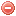# Description

The Buildup index ($$BUI$$) is one of the two intermediate indices required for calculating the Canadian fire weather index ($$FWI$$\)). The $$BUI$$ represents the total amount of fuel available to the spreading fire. It is a combination of the $$DMC$$ and the $$DC$$ (Van Wagner 1987).

The $$BUI$$ is affected mainly by the $$DMC$$. The $$DC$$ only has a limited and variable weight in the equation (Van Wagner 1987).

# Formula

For $$DMC\leqslant{0.4\cdot DC}$$ the $$BUI$$ is calculated as follows (Van Wagner and Pickett 1985):

$BUI=0.8\cdot\dfrac{DMC\cdot{DC}}{DMC+0.4\cdot DC}, \mbox{ for DMC}\leqslant{0.4\cdot DC}$

and for $$DMC>{0.4 \cdot DC}$$ as follows:

$BUI=DMC-\bigg(1-\dfrac{0.8\cdot DC}{DMC+0.4\cdot DC}\bigg)\cdot\big[0.92+(0.0114\cdot DMC)^{1.7}\big], \mbox{ for DMC}>{0.4\cdot DC}$

where $$DMC$$ is the Duff moisture code and $$DC$$ the Drought code.

Original publication:
Van Wagner and Pickett (1985)
Van Wagner (1987)

### Bibliography SearchNo results!

No results were found. Are you sure you searched for a tag?

### Symbols

 Variable Description Unit $$T$$ air temperature °C $$T_{dew}$$ dew point temperature °C $$H$$ air humidity % $$P$$ rainfall mm $$U$$ windspeed m/s $$w$$ days since last rain (or rain above threshold) d $$rr$$ days with consecutive rain d $$\Delta t$$ time increment d $$\Delta{e}$$ vapor pressure deficit kPa $$e_s$$ saturation vapor pressure kPa $$e_a$$ actual vapor pressure kPa $$p_{atm}$$ atmospheric pressure kPa $$PET$$ potential evapotranspiration mm/d $$r$$ soil water reserve mm $$r_s$$ surface water reserve mm $$EMC$$ equilibrium moisture content % $$DF$$ drought factor - $$N$$ daylight hours hr $$D$$ weighted 24-hr average moisture condition hr $$\omega$$ sunset hour angle rad $$\delta$$ solar declination rad $$\varphi$$ latitude rad $$Cc$$ cloud cover Okta $$J$$ day of the year (1..365/366) - $$I$$ heat index - $$R_n$$ net radiation MJ⋅m-2⋅d-1 $$R_a$$ daily extraterrestrial radiation MJ⋅m-2⋅d-1 $$R_s$$ solar radiation MJ⋅m-2⋅d-1 $$R_{so}$$ clear-sky solar radiation MJ⋅m-2⋅d-1 $$R_{ns}$$ net shortwave radiation MJ⋅m-2⋅d-1 $$R_{nl}$$ net longwave radiation MJ⋅m-2⋅d-1 $$\lambda$$ latent heat of vaporization MJ/kg $$z$$ elevation m a.s.l. $$d_r$$ inverse relative distance Earth-Sun - $$\alpha$$ albedo or canopy reflection coefficient - $$\Delta$$ slope of the saturation vapor pressure curve kPa/°C $$Cc$$ cloud cover eights $$ROS$$ rate of spread m/h $$RSF$$ rate of spread factor - $$WF$$ wind factor - $$WRF$$ water reserve factor - $$FH$$ false relative humidity - $$FAF$$ fuel availability factor - $$PC$$ phenological coefficient -

 Suffix Description $$-$$ mean / daily value $$_{max}$$ maximum value $$_{min}$$ minimum value $$_{12}$$ value at 12:00 $$_{13}$$ value at 13:00 $$_{15}$$ value at 15:00 $$_{m}$$ montly value $$_{y}$$ yearly value $$_{f/a}$$ value at fuel-atmosphere interface $$_{dur}$$ duration $$_{soil}$$ value at soil level

 Constant Description $$e$$ Euler's number $$\gamma$$ psychrometric constant $$G_{SC}$$ solar constant $$\sigma$$ Stefan-Bolzmann constant